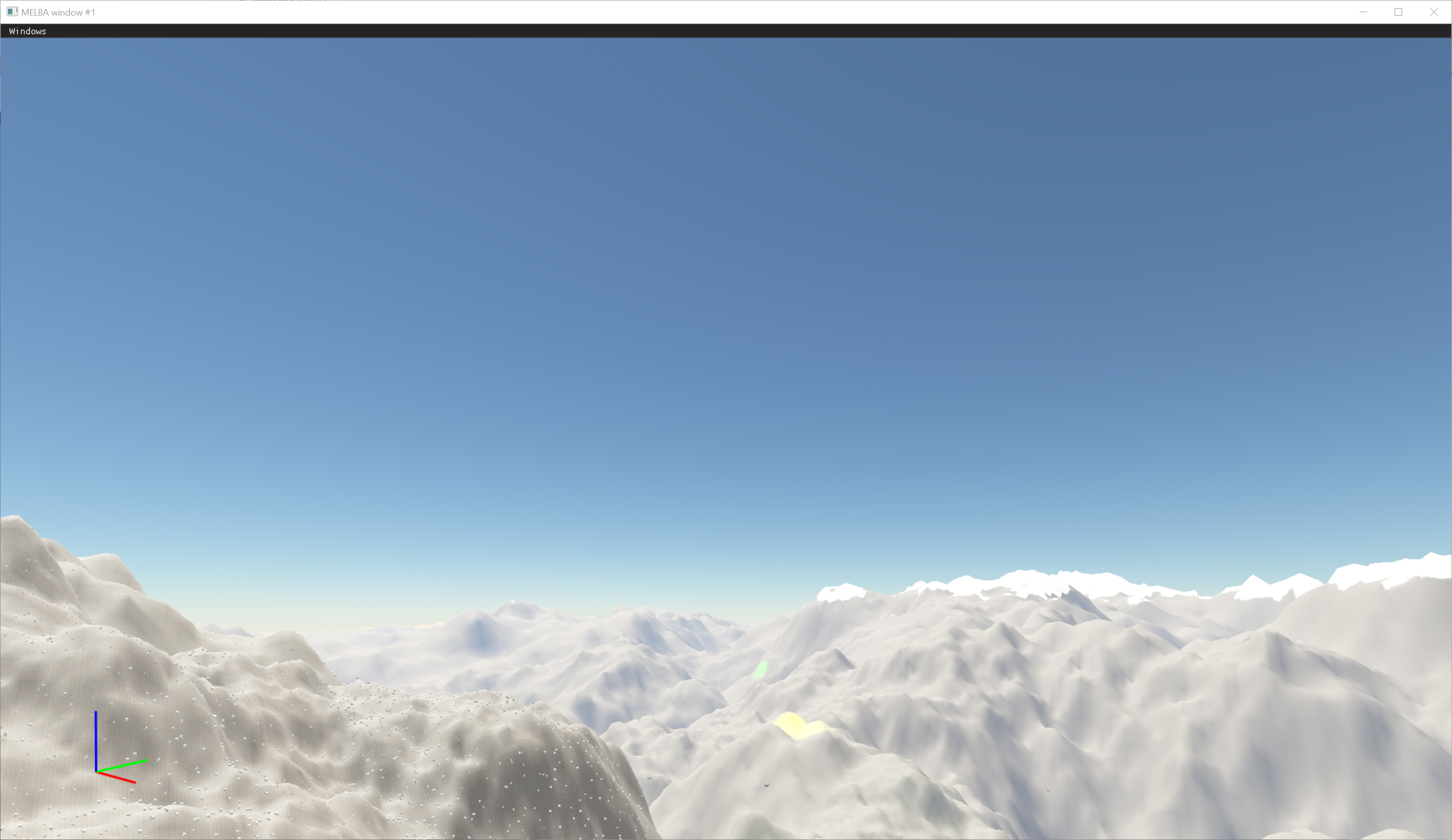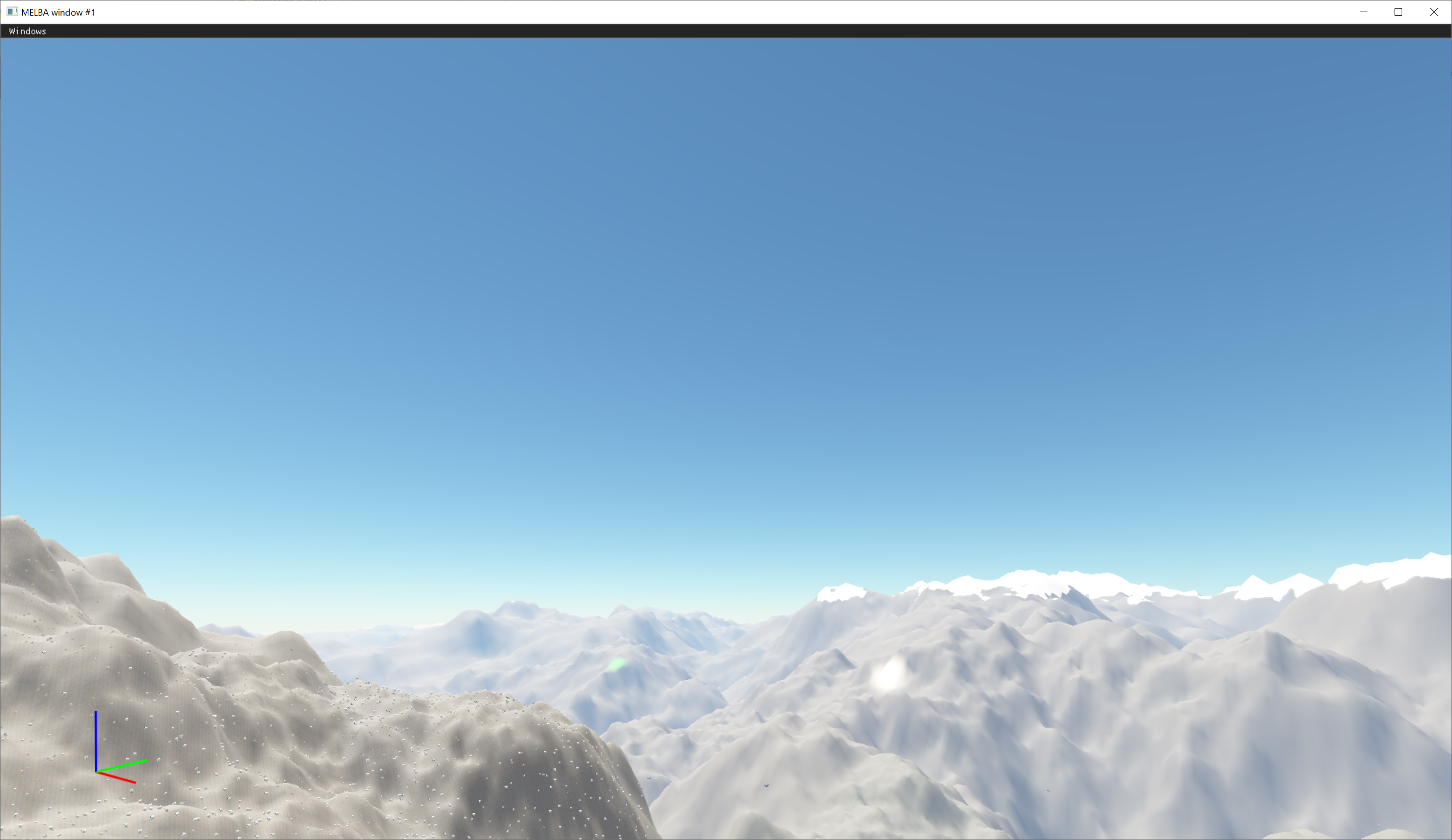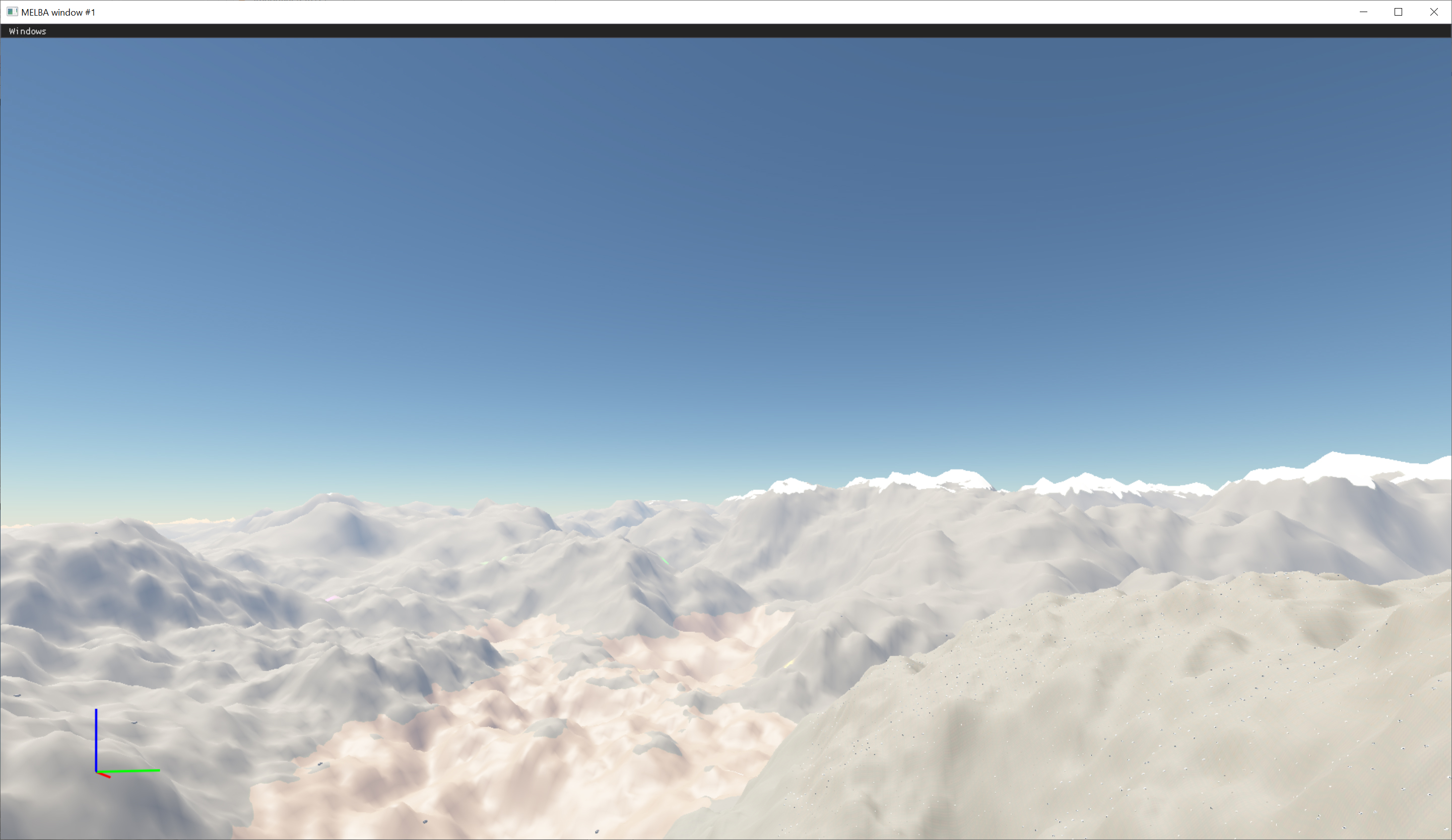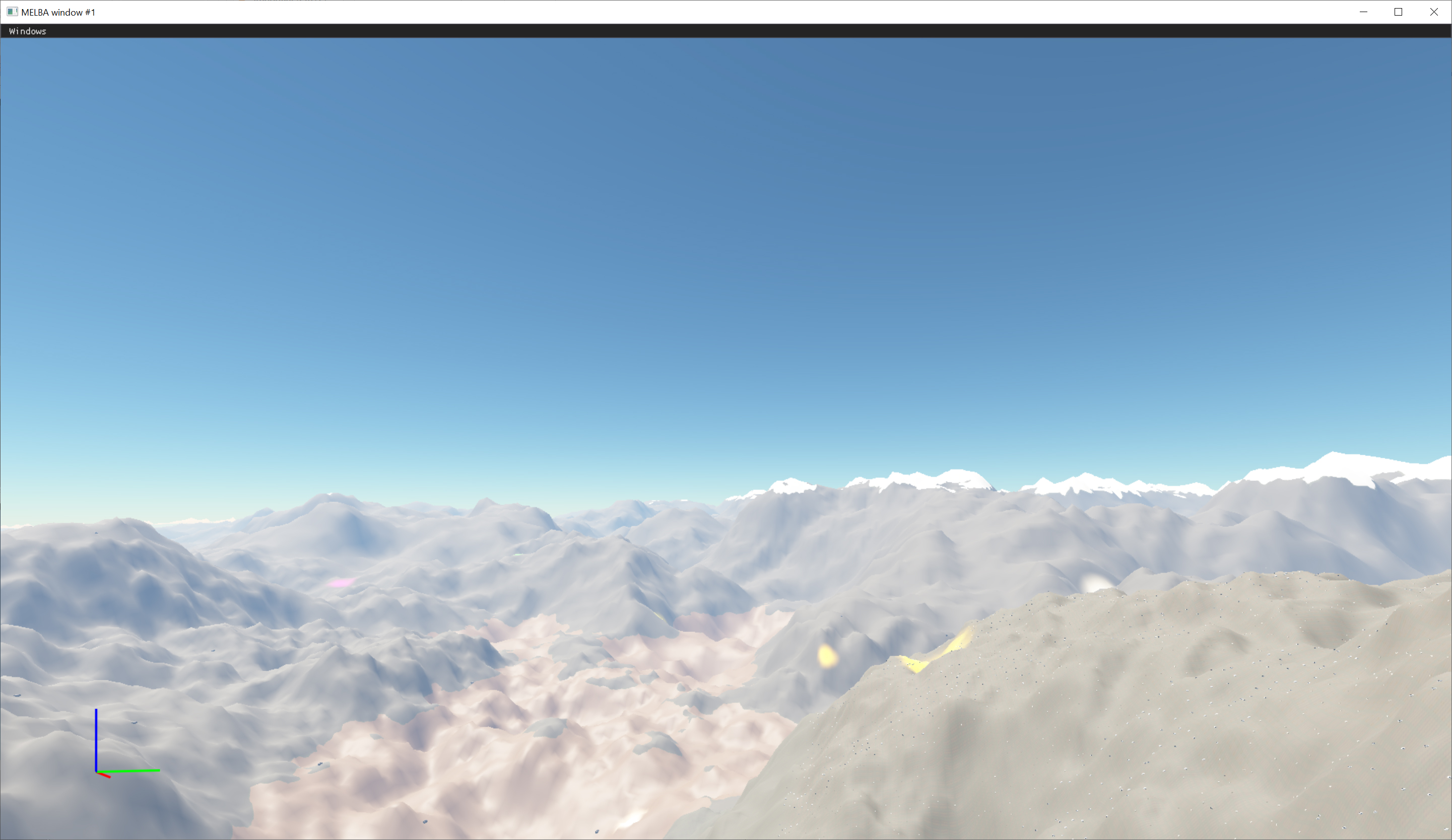Figure 8: Nice views of the sundisk

Finally, we use a configurable extra-terrestrial solar irradiance with 3 constants . We multiply 3 spectrum samples L(λr), L(λg), and L(λb) by these 3 constants to get the RGB color. This gives us a relatively accurate approximation compared to spectral rendering with a large number of wavelengths . See the difference between before and after in Figure 9.

* In computer graphics, spectral rendering is a technique in which a scene's light transport is modelled with actual wavelengths .Figure 9a: Before & after spectral renderingFigure 9b: Before & after spectral rendering

## Future improvements

The next iteration aims to add multiple scattering, and some parameter values (such as the thickness of the atmosphere) may be adjusted. We also plan to extend the method to support non-opaque objects (transparent, volumetric, etc.).

For the challenges/features we see in the future, we need to think about how to integrate the weather with atmospheric scattering on our planet. I assume this step will be challenging.

If you are interested in joining us to tackle these challenges or even invent an entirely novel solution, then check out our career page.

## Reference

 Nishita 1993, Display of The Earth Taking into Account Atmospheric Scattering -

http://nishitalab.org/user/nis/cdrom/sig93_nis.pdf

 Bruneton & Neyret 2008, Precomputed Atmospheric Scattering - https://hal.inria.fr/inria-00288758/document

 Angular Diameter - https://www.astronomy.swin.edu.au/cosmos/A/Angular+Diameter

 Precomputed Atmospheric Scattering: a New Implementation - https://ebruneton.github.io/precomputed_atmospheric_scattering/

 A Qualitative and Quantitative Evaluation of 8 Clear Sky Models - https://arxiv.org/pdf/1612.04336.pdf

 Spectral rendering - Spectral rendering

## Appendix A

Reconstruct position from UV and depth

```float3 get_world_space_local_position(float2 p_uv, float p_depth, float4x4 p_inv_view_proj_local) { // Get position in clip space float4 l_pos_cs = float4(p_uv * 2.0 - 1.0, p_depth, 1.0); l_pos_cs.y = -l_pos_cs.y; // Get position in camera local world space float4 l_pos_ws_local = mul(l_pos_cs, p_inv_view_proj_local); return l_pos_ws_local.xyz / l_pos_ws_local.w; }```
```  ```

```
```

## Appendix B

Calculate the Rayleigh constant

```//========================================================================= // @brief Get the Rayleigh/Mie particle polarisability constant // @param p_n, The index of refraction of the atmosphere at sea level // @param p_ns, The Rayleigh/Mie particles' molecular number density of the atmosphere at sea level // @ref [Nishita 1993, Display of The Earth Taking into Account Atmospheric Scattering] : Equation 1 float get_particle_polarisability_constant(float p_n, float p_ns) { float l_n2_minus_1 = p_n * p_n - 1.0f; float l_k = 2.0f * M_PI * M_PI * l_n2_minus_1 * l_n2_minus_1 / 3.0f / p_ns; return l_k; }```
```  ```

```
```

Calculate the Rayleigh scattering coefficient

```//========================================================================= // @brief Get the Rayleigh scattering coefficient // @param p_k, The Rayleigh particle polarisability constant // @param p_wavelength, The wavelength of visible light // @ref [Nishita 1993, Display of The Earth Taking into Account Atmospheric Scattering] : Equation 3 mb_math::float3 get_rayleigh_scattering_coefficient(float p_k, const mb_math::float3& p_wavelength) { mb_math::float3 l_wavelength4(pow(p_wavelength.x, 4.0f), pow(p_wavelength.y, 4.0f), pow(p_wavelength.z, 4.0f)); float l_numerator = 4.0f * M_PI * p_k; return mb_math::float3(l_numerator / l_wavelength4.x, l_numerator / l_wavelength4.y, l_numerator / l_wavelength4.z); }```
```  ```

```
```

## Appendix C

Ray sphere intersection

```//----------------------------------------------------------------------------- // Return the scale of the ray direction (near, far) float2 ray_sphere_intersect(float3 p_ray_origin, float3 p_ray_dir, float3 p_sphere_center, float p_sphere_radius) { p_ray_origin -= p_sphere_center; float l_a = dot(p_ray_dir, p_ray_dir); float l_b = 2.0f * dot(p_ray_origin, p_ray_dir); float l_c = dot(p_ray_origin, p_ray_origin) - p_sphere_radius * p_sphere_radius; float l_d = l_b * l_b - 4.0f * l_a * l_c; if (l_d < 0) { return -1; } else { l_d = sqrt(l_d); return float2(-l_b - l_d, -l_b + l_d) / (2.0f * l_a); } }```
```  ```

```
```

## Appendix D

Get the path to ray march on the 1st ray

```// Get initial attributes of the ray float3 l_ray_start = l_camera.m_camera_pos; float3 l_ray_dir = normalize(l_pos_ws_local); float l_ray_length = length(l_pos_ws_local); // Get intersections with the outer atmosphere (near, far) float2 l_intersections = ray_sphere_intersect(l_ray_start, l_ray_dir, g_push_constants.m_planet_center, g_push_constants.m_planet_radius + g_push_constants.m_atmosphere_height); // Get starting point and end point of the ray float l_ray_start_scale = max(l_intersections.x, 0.0f); float l_ray_end_scale = min(l_intersections.y, l_ray_length); // Return if there is no intersection with the atmosphere or the ray is invalid if (l_ray_start_scale >= l_ray_end_scale) { l_rt[p_dispatch_thread_id.xy].xyz = pack_lighting(get_sun_disc_mask(l_light_dir, l_ray_dir) * l_light_color); return; }```
```  ```

```
```

## Appendix E

Get optical depth along the light direction

```//----------------------------------------------------------------------------- // Get optical depth along the light direction float2 get_optical_depth_along_light_direction(float3 p_ray_start, float3 p_ray_dir, float3 p_planet_center, float p_planet_radius, float p_atmosphere_height, float2 p_density_scale_height, uint p_sample_count) { // Get the intersection with the planet float2 l_intersection = ray_sphere_intersect(p_ray_start, p_ray_dir, p_planet_center, p_planet_radius); // Intersect with the planet if (l_intersection.x > 0) { return 1e20; } // Get the intersection with the outer atmosphere l_intersection = ray_sphere_intersect(p_ray_start, p_ray_dir, p_planet_center, p_planet_radius + p_atmosphere_height); float l_ray_end = l_intersection.y; // Compute the optical depth along the ray float l_step_size = l_ray_end / p_sample_count; float2 l_optical_depth = 0.0f; for (uint l_i = 0; l_i < p_sample_count; l_i++) { // Sample in the middle of the segment float3 l_position = p_ray_start + (l_i + 0.5f) * l_step_size * p_ray_dir; float2 l_density_ratio = get_density_ratio(l_position, p_planet_center, p_planet_radius, p_density_scale_height); l_optical_depth += l_density_ratio * l_step_size; } return l_optical_depth; }```
```  ```

```
```

## Appendix F

Phase function

```//----------------------------------------------------------------------------- // Phase function for Rayleigh scattering // [Nishita 1993, Display of The Earth Taking into Account Atmospheric Scattering] : Equation 5 // We divided by an extra 4 pi since we want to use the scattering coefficient in the final scattering equation float phase_function_rayleigh(float p_cos_theta) { return (3.0f / (16.0f * M_PI)) * (1 + p_cos_theta * p_cos_theta); } //----------------------------------------------------------------------------- // Phase function for Mie scattering // Cornette-Shanks phase function // [Nishita 1993, Display of The Earth Taking into Account Atmospheric Scattering] : Equation 5 // We divided by an extra 4 pi since we want to use the scattering coefficient in the final scattering equation float phase_function_mie(float p_cos_theta, float p_mie_g) { float l_mie_g2 = p_mie_g * p_mie_g; return 1.5f * 1.0f / (4.0f * M_PI) * (1.0f - l_mie_g2) * (1.0f + p_cos_theta * p_cos_theta) * pow(1.0f + l_mie_g2 - 2.0f * p_mie_g * p_cos_theta, -3.0f / 2.0f) / (2.0f + l_mie_g2); }```
```  ```

```
```

## Appendix G

Combine everything and get the final scattering result

```// Calculate the in-scattering float3 l_light_inscattering = (l_integrated_inscattering_rayleigh * g_push_constants.m_rayleigh_scattering_coefficient + l_integrated_inscattering_mie * g_push_constants.m_mie_scattering_coefficient) * g_push_constants.m_solar_irradiance * l_light_color; // Calculate the out-scattering (without reflected light from earth) float3 l_light_extinction = exp(-(l_optical_depth_camera_to_point.x * g_push_constants.m_rayleigh_scattering_coefficient + l_optical_depth_camera_to_point.y * g_push_constants.m_mie_scattering_coefficient)); float3 l_lighting = unpack_lighting(l_rt[p_dispatch_thread_id.xy].xyz); // Final scattering result // [Nishita 1993, Display of The Earth Taking into Account Atmospheric Scattering] : Equation 9 l_lighting = l_lighting * l_light_extinction + l_light_inscattering;```
```  ```

```
```

## Appendix H

Generate lookup table

```// UV to altitude and cosine theta float l_altitude = l_uv.x * g_push_constants.m_atmosphere_height; float l_cos_theta = -1.0f + l_uv.y * 2.0f; // Get density ratio of current point float2 l_density_ratio = exp(-l_altitude.xx / g_push_constants.m_density_scale_height); // Get optical depth along the light direction float3 l_ray_start = float3(0.0f, l_altitude + g_push_constants.m_planet_radius, 0.0f); float3 l_ray_dir = normalize(float3(sqrt(1.0f - l_cos_theta * l_cos_theta), l_cos_theta, 0.0f)); float2 l_optical_depth = get_optical_depth_along_light_direction(l_ray_start, l_ray_dir, float3(0.0f, 0.0f, 0.0f), g_push_constants.m_planet_radius, g_push_constants.m_atmosphere_height, g_push_constants.m_density_scale_height, g_push_constants.m_sample_count); l_rt[p_dispatch_thread_id.xy] = float4(l_density_ratio, l_optical_depth);```
```  ```

```
```